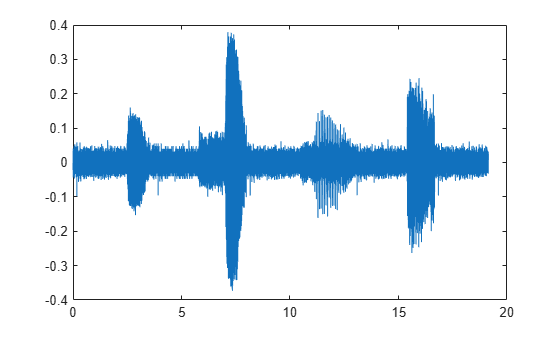Add members to labeled signal set

## Syntax

``addMembers(lss,src)``
``addMembers(lss,src,tinfo)``
``addMembers(lss,src,tinfo,mnames)``

## Description

example

````addMembers(lss,src)` adds members to the labeled signal set `lss` from the input data source `src`.```
````addMembers(lss,src,tinfo)` sets the time information for the new members to `tinfo`.```
````addMembers(lss,src,tinfo,mnames)` sets the names of the new members to `mnames`. The length of `mnames` must be equal to the number of new members.```

## Examples

collapse all

Load a labeled signal set containing recordings of whale songs.

```load whales lss```
```lss = labeledSignalSet with properties: Source: {2x1 cell} NumMembers: 2 TimeInformation: "sampleRate" SampleRate: 4000 Labels: [2x3 table] Description: "Characterize wave song regions" Use labelDefinitionsHierarchy to see a list of labels and sublabels. Use setLabelValue to add data to the set. ```

Retrieve the second member of the set and plot it.

```[song,tinfo] = getSignal(lss,2); t = (0:length(song)-1)/tinfo.SampleRate; plot(t,song)```Remove the first and last seconds of the retrieved signal.

```song2 = song(t>1 & t<t(end)-1); t2 = (0:length(song2)-1)/tinfo.SampleRate; plot(t2,song2)```Add the shorter signal as a new member of the labeled set.

```addMembers(lss,song2) lss```
```lss = labeledSignalSet with properties: Source: {3x1 cell} NumMembers: 3 TimeInformation: "sampleRate" SampleRate: 4000 Labels: [3x3 table] Description: "Characterize wave song regions" Use labelDefinitionsHierarchy to see a list of labels and sublabels. Use setLabelValue to add data to the set. ```

Flip the shorter signal upside-down and add it as a new member of the labeled set. Specify that the new member is sampled at 1 kHz.

```addMembers(lss,flipud(song2),1000) lss.SampleRate```
```ans = 4×1 4000 4000 4000 1000 ```

## Input Arguments

collapse all

Labeled signal set, specified as a `labeledSignalSet` object.

Example: ```labeledSignalSet({randn(100,1) randn(10,1)},signalLabelDefinition('female'))``` specifies a two-member set of random signals containing the attribute `'female'`.

Input data source, specified as a matrix, a cell array, a timetable, a `signalDatastore` object, or an `audioDatastore` (Audio Toolbox) object. The particular form of `src` depends on the Source property of `lss`.

• If Source is a cell array of matrices:

• Specify `src` as a matrix to add one member to the set.

• Specify `src` as a cell array of matrices to add multiple members to the set.

• If Source is a cell array containing cell arrays of vectors:

• Specify `src` as a cell array of vectors to add one member to the set.

• Specify `src` as a cell array containing cell arrays of vectors to add multiple members to the set.

• If Source is a cell array of timetables:

• Specify `src` as a timetable to add one member to the set.

• Specify `src` as a cell array of timetables to add multiple members to the set.

• If Source is a datastore, then add members by setting `src` as another datastore that points to new files.

Example: `{randn(10,3),randn(17,9)}` specifies two members. The first member contains three 10-sample signals. The second member contains nine 17-sample signals.

Example: `{{randn(10,1)},{randn(17,1),randn(27,1)}}` specifies two members. The first member contains one 10-sample signal. The second member contains a 17-sample signal and a 27-sample signal.

Example: `{{timetable(seconds(1:10)',randn(10,3)),timetable(seconds(1:7)',randn(7,2))},{timetable(seconds(1:3)',randn(3,1))}}` specifies two members. The first member contains three signals sampled at 1 Hz for 10 seconds and two signals sampled at 1 Hz for 7 seconds. The second member contains one signal sampled at 1 Hz for 3 seconds.

#### Example: `signalDatastore` Object Pointing to Files

Specify the path to a set of sample sound signals included as MAT-files with MATLAB®. Each file contains a signal variable and a sample rate. List the names of the files.

```folder = fullfile(matlabroot,"toolbox","matlab","audiovideo"); lst = dir(append(folder,"/*.mat")); nms = {lst(:).name}'```
```nms = 7x1 cell {'chirp.mat' } {'gong.mat' } {'handel.mat' } {'laughter.mat'} {'mtlb.mat' } {'splat.mat' } {'train.mat' } ```

Create a signal datastore that points to the specified folder. Set the sample rate variable name to `Fs`, which is common to all files. Generate a subset of the datastore that excludes the file `mtlb.mat`, which differs from the other files in that the signal variable is not called `y`.

```sds = signalDatastore(folder,"SampleRateVariableName","Fs"); sdss = subset(sds,~strcmp(nms,"mtlb.mat"));```

Use the subset datastore as the source for a `labeledSignalSet` object.

`lss = labeledSignalSet(sdss)`
```lss = labeledSignalSet with properties: Source: [1x1 signalDatastore] NumMembers: 6 TimeInformation: "inherent" Labels: [6x0 table] Description: "" Use labelDefinitionsHierarchy to see a list of labels and sublabels. Use setLabelValue to add data to the set. ```

Time information for new members, specified as a scalar, a vector, a matrix, a duration scalar, or a duration vector. This argument is valid only if the TimeInformation property of `lss` is `'sampleRate'`, `'sampleTime'`, or `'timeValues'`.

• If TimeInformation is `'sampleRate'`, then `tinfo` specifies sample rate values.

• If TimeInformation is `'sampleTime'`, then `tinfo` specifies sample time values.

• If TimeInformation is `'timeValues'`, then `tinfo` specifies time values.

If you add multiple members to a set, then specifying only one value of `tinfo` sets the same value for all members. If you want to specify a different value for each new member, then set `tinfo` to have multiple values.

When no source has been specified, or when the labeled signal set source is empty, you can change the TimeInformation property to `'sampleRate'`, `'sampleTime'`, or `'timeValues'` to make `lss` interpret `tinfo` correctly.

Example: ```addMembers(ks,{randn(10,5),randn(10,3)},seconds([1 2]))``` adds two new members with different time information to ```ks = labeledSignalSet(randn(10,3),'SampleTime',seconds(1))```.

Example: `addMembers(ks,{randn(10,5),randn(10,3)},[1:10;2:2:20]')` adds two new members with different time information to ```ks = labeledSignalSet(randn(10,3),'TimeValues',1:10)```.

Member names, specified as a character vector, a string scalar, a cell array of character vectors, or a string array.

Example: ```labeledSignalSet({randn(100,1) randn(10,1)},'MemberNames',{'llama' 'alpaca'})``` specifies a set of random signals with two members, `'llama'` and `'alpaca'`.

## Version History

Introduced in R2018b